# Float to integer rounding on PowerPC

cancel
Showing results for
Did you mean:
SOLVED

## Float to integer rounding on PowerPC

1,268 Views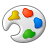Contributor V

Hi,

We've used "efsctsi" assembly instruction on MPC5xxx MCU and "fctiw" on MPC83xx PowerQUICC II Pro CPU (having SPEFSCR[FRMC] / FPSCR[RN] = 00 (round to nearest)) and we were surprised of the result. We were expecting the following 32bits floats to be converted to the following integers:

. 6.5 -> expected=7 ; obtained=6

. 7.5 -> expected=8 (obtained that)

. 8.5 -> expected=9 ; obtained=8

. -6.5 -> expected = -7 ; obtained = -6

. -7.5 -> expected = -8 (obtained that)

. -8.5 -> expected = -9 ; obtained = -8

So, is it possible to use these instructions to round floats to integer including round +x.5 to x+1 and -x.5 to -x-1? Or are these instructions performing a base-2 rounding rather than a base-10 rounding?

Thanks.

Labels (1)
• ### General

Tags (6)
1 Solution
607 ViewsNXP TechSupport

I can confirm observed behavior. I have test with e200z759. It is mode "Round to nearest, ties to even" and it seems to be fixed on this core.

IEEE floating point - Wikipedia, the free encyclopedia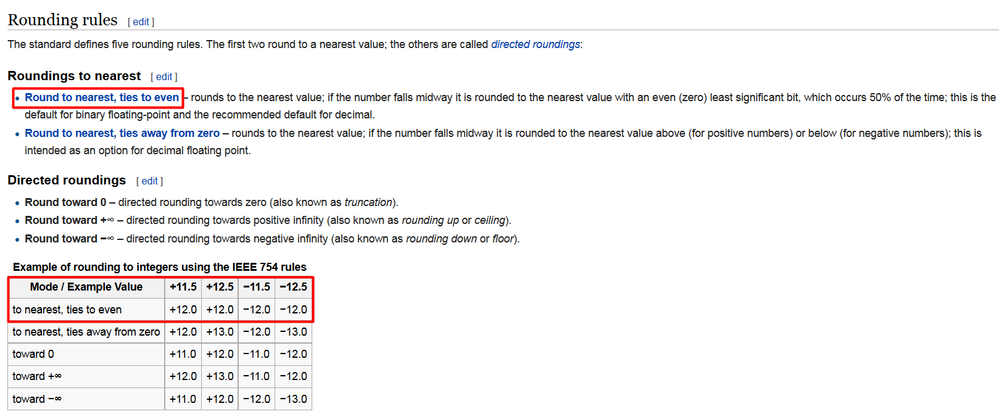e200z760 has following SPEFSCR[RM] affecting rounding mode. Note that FRMC bit also influence float to integer rounding behavior so here you should be able to configure all options from table above.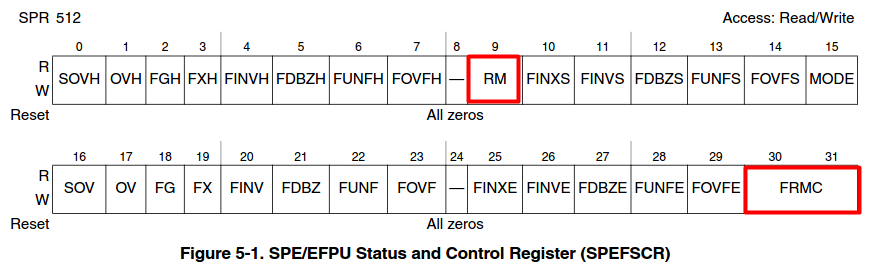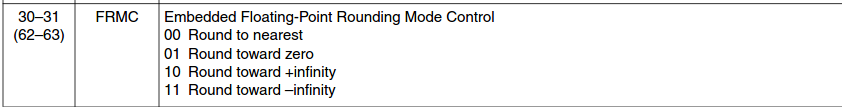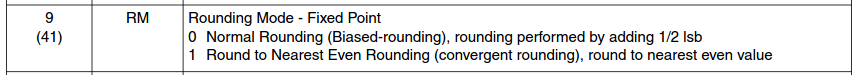6 Replies
607 ViewsNXP TechSupport

I believe it’ll have something to do with hexadecimal representation of float number as this number is rounded up/down/nearest (not its decimal interpretation) if result of calculation is inexact.

I have found in some old response following example I think could fit to this situation:

When we do calculation 5.0/3.0 = 1.66666 (round to the nearest)

The actual result is 1.66666 (in HEX 0x3FD55555) although assumption could be 1.66667

Possible a single-precision HEX result are:

0x3fd55554

0x3fd55555

0x3fd55556

and converting these possibilities to decimal are:

1.66666651

1.66666663

1.66666675

1.66666663 is the closest representation to the actual number.

607 ViewsContributor V

Hi,

I think you are discussing floating-point number rounding for floating-point operations such as multiplication or division. The PowerPC manuals have doog info on this. However, "efsctsi" / "fctiw" are different. They round the floating-point number to its nearest _integer_, thus we are not discussing rounding of the very last digit of a number, but rounding of all the fractional digits into the lowest integral digit. And this process is not well documented. There is indeed a pseudo code in the annexes of the EREF, but hard to understand... And still, I don't understand why even numbers are wrongly rounded (6.5, 8.5, 10.5) whereas odd numbers are ok (7.5, 9.5, 11.5).

Thanks.

607 ViewsNXP TechSupport

Could you give me hexadecimal representation of mentioned floating values?

607 ViewsContributor V

For example, 6.5 is 0x40D00000. And it is rounded to integer 0x00000006.

608 ViewsNXP TechSupport

I can confirm observed behavior. I have test with e200z759. It is mode "Round to nearest, ties to even" and it seems to be fixed on this core.

IEEE floating point - Wikipedia, the free encyclopediae200z760 has following SPEFSCR[RM] affecting rounding mode. Note that FRMC bit also influence float to integer rounding behavior so here you should be able to configure all options from table above.607 ViewsContributor V

Thanks. So if using e200z760 (which is used in very few MCUs though...), then setting SPEFSCR[RM]=0 and SPEFSCR[FRMC]=00 would fix the problem.